Courses

# Test: Diode Circuits

## 10 Questions MCQ Test Electronic Devices | Test: Diode Circuits

Description
This mock test of Test: Diode Circuits for Electrical Engineering (EE) helps you for every Electrical Engineering (EE) entrance exam. This contains 10 Multiple Choice Questions for Electrical Engineering (EE) Test: Diode Circuits (mcq) to study with solutions a complete question bank. The solved questions answers in this Test: Diode Circuits quiz give you a good mix of easy questions and tough questions. Electrical Engineering (EE) students definitely take this Test: Diode Circuits exercise for a better result in the exam. You can find other Test: Diode Circuits extra questions, long questions & short questions for Electrical Engineering (EE) on EduRev as well by searching above.
QUESTION: 1

### For the given circuits and input waveform determine the output waveform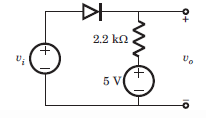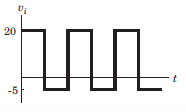Solution:

Diode is off when Vi < 5 and for Vi > 5, Vo = 5V.

QUESTION: 2

### For the given circuits and input waveform determine the output waveform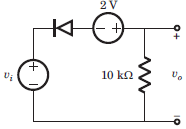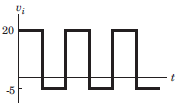Solution:

Diode is off when Vi + 2 > 0.

QUESTION: 3

### For the given circuits and input waveform determine the output waveform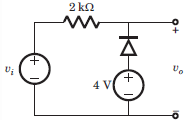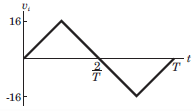Solution:

For V1 < 4 the diode is on otherwise its off.

QUESTION: 4

For the given circuits and input waveform determine the output waveform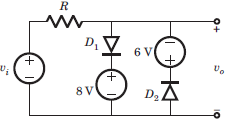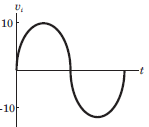Solution:

During +ve half cycle when Vi < 8 both the diodes are off. For V1 > 8, D1 is on. Similarly for the negative half cycle.

QUESTION: 5

The maximum load current that can be drawn is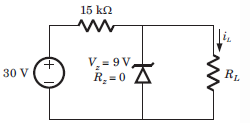Solution:

At regulated poer supply, Is = 30-9/15k or 1.4 mA. The load current will remain less than this current.

QUESTION: 6

For the circuit shown diode cutting voltage is Vin = 0. The ripple voltage is to be no more than vrip = 4 V. The minimum load resistance, that can be connected to the output is (in kilo ohm)​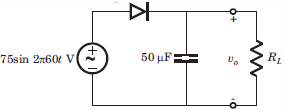Solution: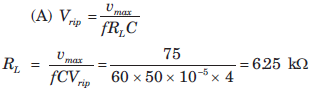QUESTION: 7

The circuit inside the box in fig. P3.1.31. contains only resistor and diodes. The terminal voltage vo isconnected to some point in the circuit inside the box. The largest and smallest possible value of vo most nearly to is respectively​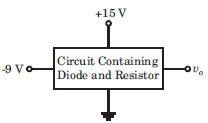Solution:

The output voltage cannot exceed the positive power supply voltage and cannot be lower than the negative power supply voltage.

QUESTION: 8

The Q-point of the zener diode in the circuit shown below is​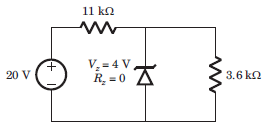Solution: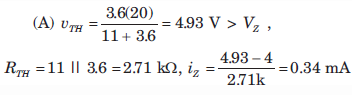QUESTION: 9

A 2-port network is driven by a source Vs = 100 V in series with 5 ohm, and terminated in a 25 ohm resistor. The impedance parameters are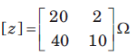The Thevenin equivalent circuit presented to the 25 ohm resistor is

Solution:

100 = 25I1 + 2I2, V2 = 40I1 + 10I2
Also V2 = 160I1 + 6.8I2
using above equations we get, Vth = 160 V and Rth = 6.8 ohm.

QUESTION: 10

Find V1 and V2.​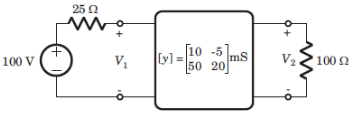Solution:

800 = 10V1 – V2 and 3V2 = 5V1 = 0.